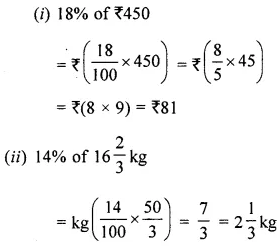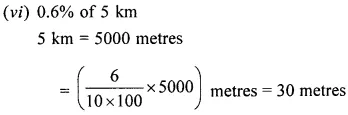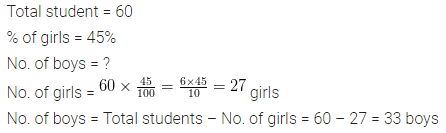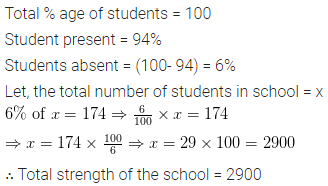ML Aggarwal Class 6 Solutions Chapter 8 Ratio and Proportion Ex 8.4 for ICSE Understanding Mathematics acts as the best resource during your learning and helps you score well in your exams.

## ML Aggarwal Class 6 Solutions for ICSE Maths Chapter 8 Ratio and Proportion Ex 8.4

Question 1.
Find the value of:Solution:Question 2.
In a class of 60 student, 45% are girls. Find the number of boys in the class.
Solution:Question 3.
Mr. Malkani saves 22% of his salary every month. If his salary is ₹ 12750 per month, what is his expenditure?
Solution:Question 4.
On a rainy day, 94% of the students were present in a school, if the number of students absent on that day was 174, find the total strength of the school.
Solution: